# High School Math : Using the Quadratic Formula

## Example Questions

### Example Question #1 : Using The Quadratic Formula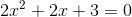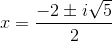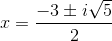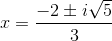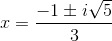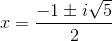Explanation:

Use the quadratic formula to solve: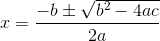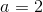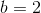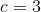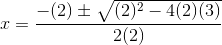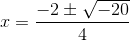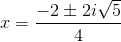### Example Question #2 : Using The Quadratic Formula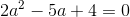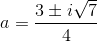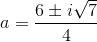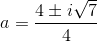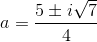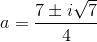Explanation:

Use the quadratic formula to solve: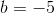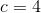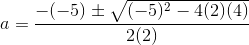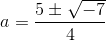### Example Question #3 : Using The Quadratic Formula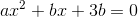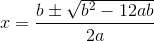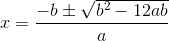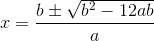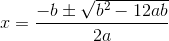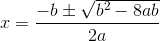Explanation:

Use the quadratic formula to solve: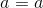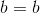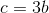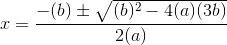### Example Question #4 : Using The Quadratic Formula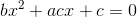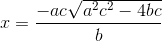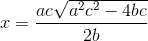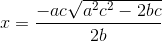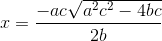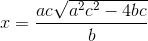Explanation:

Use the quadratic formula to solve: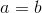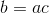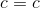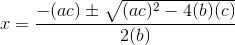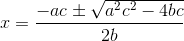### Example Question #5 : Using The Quadratic Formula

A baseball that is thrown in the air follows a trajectory of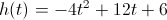, where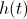is the height of the ball in feet andis the time elapsed in seconds. How long does the ball stay in the air before it hits the ground?

Between 3 and 3.5 seconds

Between 3.5 and 4 seconds

Between 2 and 2.5 seconds

Between 4 and 4.5 seconds

Between 2.5 and 3 seconds

Between 3 and 3.5 seconds

Explanation:

To solve this, we look at the equation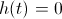.

Setting the equation equal to 0 we get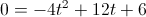.

Once in this form, we can use the Quadratic Formula to solve for.

The quadratic formula says that if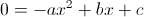, then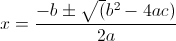.

Plugging in our values: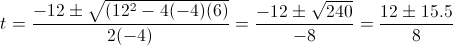Therefore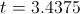or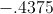and since we are looking only for positive values (because we can't have negative time), 3.4375 seconds is our answer.